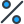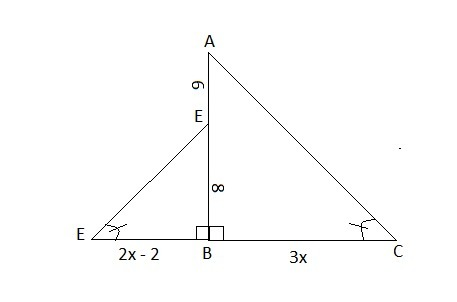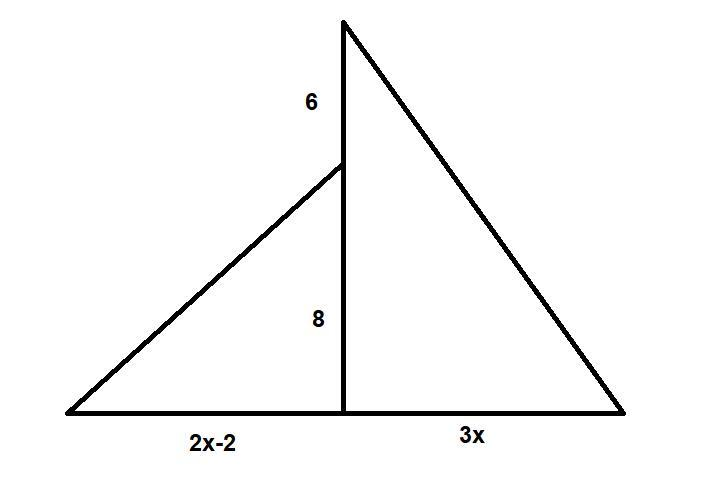# The two triangles are similar. What is the value of x? Enter your answer in the box. x = Two right triangles, one smaller than the other, back to back, so that their right angles form a straight angle. The two acute angles along the straight angle are congruent to each other. The overlapping part of the legs is labeled 8. The part of the overlapping side that extends above the smaller triangle is labeled 6. The leg of the smaller triangle that is a ray of the straight angle is labeled 2 x minus 2. The leg of the larger angle that is a ray of the straight angle is labeled 3 x.

•Subject:

Mathematics
• Author:

laytonmcintosh
• Created:

7 months ago

x = 7

Step-by-step explanation:

For better understanding of the solution see the attached figure :

Given : AD = 6 units , BD = 8 units, m∠ABC = m∠DBE = 90° and               ∠DEB ≅ ∠ACB

Now, AB = AD + BD

= 8 + 6 = 14 units

In ΔABC and ΔDBE,

∠DBE = ∠ABC ( Each of 90° )

∠DEB = ∠ACB ( Both angles are congruent to each other )

So, By using AA postulate of similarity of triangles , ΔABC ~ ΔDBE

So, proportion of the corresponding sides will be equal.

\implies \frac{AB}{DB}=\frac{BC}{BE}=\frac{AC}{DE}\\\\\implies \frac{AB}{DB}=\frac{BC}{BE}\\\\\implies \frac{14}{8}=\frac{3\cdot x}{2\cdot x-2}\\\\\implies 28\cdot x-28=24\cdot x\\\implies 4\cdot x=28\\\implies x=7

Hence, x = 7The two triangles are similar. The value of x is 7.

Given information:

Two right triangles, one smaller than the other, back to back, so that their right angles form a straight angle.

The two triangles are similar.

The overlapping part of the legs is labeled 8. The part of the overlapping side that extends above the smaller triangle is labeled 6. The leg of the smaller triangle that is a ray of the straight angle is labeled 2x-2. The leg of the larger triangle that is a ray of the straight angle is labeled 3x. See the figure attached for more information.

The ratio of sides of triangles will be equal.

The ratio of the height of two triangles will be equal to the ratio of the base of them.

So, the value of x can be calculated as,

\dfrac{2x-2}{3x}=\dfrac{8}{8+6}\\\dfrac{2x-2}{3x}=\dfrac{4}{7}\\14x-14=12x\\2x=14\\x=7

Therefore, the value of x is 7.x = 10 Explanation: Since the two triangles are similar, their corresponding sides are in proportion. Therefore, we can set up a proportion using the side lengths of the smaller triangle and the larger triangle and then solve for x. The corresponding sides have the following proportion: 2x - 2 : 3x = 6 : 8 We can then solve this proportion for x: 2x - 2 = 6 ; 3x = 8 2x = 8 ; x = 4 3x = 8 ; x = 10 Therefore, the value of x is 10.

Don't worry! There are several alternative approaches you can try to resolve your query. Here are some tips to help you find answers in different ways:

1. Reframe your question: Sometimes, the way you phrase your question can limit your search results. Try rephrasing it using different keywords or providing more context to get better results.
2. Utilize social media: Post your question on social media platforms, particularly those focused on professional or specialized topics. Twitter, LinkedIn, and Facebook groups can connect you with individuals who may have relevant expertise or experiences to share.
3. Consult subject matter experts: Reach out to experts in the field related to your question. Many professionals are willing to help and share their knowledge. You can connect with them through email, LinkedIn messages, or by attending relevant conferences or events.
4. Use our website to find your question through the search box above, or you can sign up to ask your question for our big educational community. Our experts will review your question, and you will get a quick and quality answer.
5. Collaborate with others: If your question is related to a specific project or problem, consider collaborating with others who might have complementary skills or knowledge. Teamwork can lead to innovative solutions and shared insights.

Remember, the process of finding answers often involves persistence, creativity, and an open mind. By exploring various resources, reaching out to others, and being proactive in your search, you increase your chances of finding the information you need. Happy quest for knowledge!